﻿ Frequency Modulation Study of a Monofacial Solar Cells Based on Copper Indium and Gallium Diselenide (CIGS) under Monochromatic Illumination: Influence of Incidence Angle and Gallium DopingPublications are Open
Access in this journal
Article Versions
Export Article
• Normal Style
• MLA Style
• APA Style
• Chicago Style
Research Article
Open Access Peer-reviewed

### Frequency Modulation Study of a Monofacial Solar Cells Based on Copper Indium and Gallium Diselenide (CIGS) under Monochromatic Illumination: Influence of Incidence Angle and Gallium Doping

Gerome SAMBOU , Alain Kassine EHEMBA, Mouhamadou Mamour SOCE, Amadou DIAO, Moustapha DIENG
American Journal of Materials Science and Engineering. 2018, 6(1), 7-11. DOI: 10.12691/ajmse-6-1-2
Published online: April 26, 2018

### Abstract

In this article a frequency modulation study on a CIGS-based solar cells under the influence of incidence angle and gallium doping is made. The resolution of the minority carrier continuity equation allowed us to determine the density of minority carriers, the photocurrent density and photovoltage expressions according to modulation frequency, wavelength, incidence angle and gallium doping. Incidence angle and Gallium doping tend to decrease the performance of the solar cell by degrading its intrinsic properties.

### 1. Introduction

Solar cells based on Cu(In,Ga)Se2 (CIGS) have reached conversion efficiencies above 21.7% 1 in the laboratory for small areas of a few square centimetre (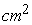). Moreover, their efficiency can be improved according to the layer synthesis method. CIGS is a direct gap semiconductor material. The CIGS gap varies according to X (the rate of gallium atoms replacing indium atoms in the structure) between the values of pure CIS and pure CGS, according to the empirical law 2: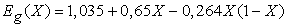Where,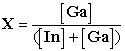3, 4

To link the characteristics of a thin-film solar cell with the properties of the CIGS material, theoretical study models have been made. Electrical parameters are determined in static mode 5, but also in dynamic mode 6 under monochromatic illumination. Thus in this work we propose a one-dimensional theoretical study in frequency modulation under monochromatic illumination on a CIGS-based monofacial solar cells model. The effects of angle of incidence and gallium doping on minority carrier density, photocurrent density and photovoltage are highlighted.

### 2. Theoretical Study

We consider a CIGS-based solar cells whose simplified structure is shown in Figure 1.

• Figure 1. Simplified schema of a CIGS-based solar cells with one dimensional dimensions

In this study, we will neglect the contribution of the emitter by focusing only on the bottom-up contribution.

Under the effect of frequency-modulated monochromatic optical excitation, minority charge (electrons) carriers are generated in the absorbing layer of the solar cells. The continuity equation of the minority carriers in the x-axis base in frequency dynamic regime is of the form: 7, 8: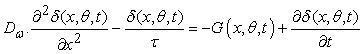(1)

With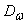the complex diffusion coefficient of minority carriers;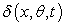the density of minority carriers;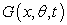the generation rate of minority carriers 9, 10 and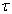the average life of minority carriers.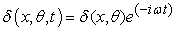(2)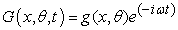(3)

Where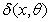and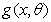are the spatial component and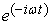is the temporal component.

For illumination from the front face of the solar cell and depending on the angle of incidence, the spatial component of the generation rate is: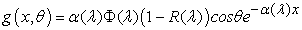(4)

Where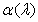is the absorption coefficient at the wavelength λ; R(λ) is the reflection coefficient of the material,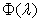the incident photon flux, and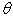the incidence angle.

By replacing equations (2), (3) and (4) in equation (1) we get: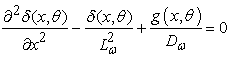(5)

with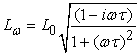(6)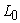intrinsic diffusion length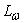the complex diffusion length

The general solution of the preceding equation (6) is given by the relation (7).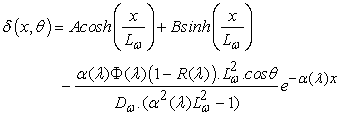(7)

The constants A and B are determined from the following boundary conditions 13:

• At junction x=0: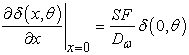(8)

• on the rear face of the base (x=H):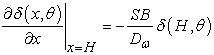(9)

SF and SB denote the recombination speeds of the minority load carriers at the junction and rear face of the base respectively.

The expression of minority carriers density is expressed as a function of the CIGS absorption coefficient. This coefficient depends on gallium doping and is given by: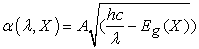with A (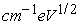) a constant 14.

Beyond the expressions of minority carriers density, photocurrent density and photovoltage are determined according to the angle of incidence of gallium doping rate, frequency and wavelength.

### 3. Results and Discussions

3.1. Density Profile of Minority Carriers

Figure 2 shows the profile of the minority carrier density module according to the base depth for different angles of incidence:

• Figure 2. Module of minority carrier density as a function of base depth for different incidence angle values θ

This Figure 2 shows that for small depth values in the base the minority carrier density module increases to a maximum corresponding to a base depth x0. Then for values of x higher than x0, the module of minority carrier density decreases. In this way, in the base of the photopile there are two zones delimited by x0:

the area where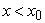In this area, the density gradient of minority carriers in the base of the photopile is positive: charge carriers located in this area can cross the junction and participate in the photocurrent. This area is considered to be an extension of depletion zone.

the area where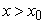In this area of the base, the module of minority carriers density decreases: the gradient is negative. Minority carriers are blocked and we obey volume and surface recombinations. These recombinations are sometimes due to the presence of deficiencies, structural defects which are positively charged, and which are mainly VSe selenium deficiencies. 15

In addition, Figure 2 also shows that the minority carriers density module decreases with increasing incidence angle. Indeed, the increase in the angle of incidence relativizes the sun's inclination according to the normal at the front face of the cell. Thus, more than one increases the angle of incidence, the greater thickness of the atmosphere is traversed by the sun and a part of radiation is absorbed. We remark a loss of energy, therefore a decrease in the density of minority carriers.

• Figure 3. Module of minority carrier density as a function of the base depth for different doping values X

In Figure 3 the four curves have the same profile. We observe the two zones (area whereand the one where) explained in the previous figure. In addition, the increase in gallium doping increases the energy of the CIGS gap and therefore the space load zone x0. Then there is a decrease in the density of minority carriers when gallium doping is increased. We observe a distance x1 of about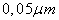above which the low-doping gallium curves are below. Thus, low doping levels favour recombinations in the depth of the base.

From the expression of minority carrier’s density, we can deduce the photocurrent density and the photovoltage respectively.

3.2. Photocurrent density profile

By applying the FICK law, at the junction of the solar cells, we obtain the density of photocurrent given by: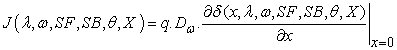(10)

q is the elementary charge of the electron.

There combinations of these electron-hole pairs in the depletion zone are among the phenomena that fundamentally limit the performance of photovoltaic devices. The speed of recombination at the junction partially relativizes the effects of these recombination phenomena.

The module profile of the photocurrent density as a function of the recombination velocity at the junction for different angle of incidence is shown in Figure 4.

• Figure 4. Photocurrent density module as a function of recombination velocity at junction for different incidence angle values

This Figure 4 shows that for large values of the recombinant junction velocity (SF) of minority carriers, the photocurrent density module tends towards a limit which is the density of the short-circuit current Jcc. For low values (SF) of the recombination velocity at the junction, there is virtually no electron flow through the junction. We're at the open circuit operating point.

In addition, we note that the density of the Jcc short-circuit current decreases when the angle of incidence is increased θ. Indeed, with the inclination of the sun (the sun is no longer at Zenith), the level of illumination decreases. This leads to a reduction in the generation of minority carriers.

The profile of the photocurrent density module as a function of the recombination velocity at the junction for different gallium doping rate is shown in Figure 5:

• Figure 5. Photocurrent density module as a function of recombination velocity at junction for different doping values X

As shown in Figure 4, for low values of the recombination speed of minority carriers at the junction, the photocurrent density module remains very low and then increases until a constant maximum value corresponding to the short circuit photocurrent is reached. Thus, we find that the more we increase X, the lower the short circuit current density Jcc decreases. Indeed, Zunger et al. suggested that changing the defect energies of generated pairs remains a possible explanation of the effects of gallium doping on the photocurrent density module 16.

From these two previous figures we can see that the short circuit current density Jcc is more affected by the angle of incidence.

3.3. Photovoltage profile

The photovoltage is deduced from the density of minority carriers using the Boltzmann relation: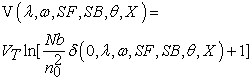(11)

with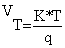;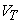is the thermal voltage, T is the absolute temperature at thermal equilibrium; k is the Boltzmann constant;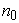is the density of the intrinsic carriers in the base and

Nb is the doping rate of impurities in the base.

In Figure 6, the photovoltage module is shown as a function of the recombination velocity at the junction, for different angles of incidence and gallium doping respectively:

• Figure 6. Profile of the photovoltage module as a function of the recombination velocity at the junction for different angle of incidence values

The Figure 6 and Figure 7 show that for low SF values the photovoltage is maximum. This range corresponds to the open circuit voltage Vco. Thereafter, if one tends towards the high values of SF, the photovoltage gradually decreases and tends towards very small values. This domain corresponding to the short circuit. Figure 6 shows a slight decrease of the photovoltage module with the increase of the incidence angle. Figure 7 shows that the photovoltage module increases with increasing gallium doping.

• Figure 7. Profile of the photovoltage module according to the recombination speed of the carriers at the junction for different doping values X

### 4. Conclusion

This article presents the results of the study of a CIGS-based solar cells, in frequency modulation, under monochromatic illumination with the influences of incidence angle and Gallium doping on minority carrier density, photocurrent density and photovoltage. The increase in incidence angle and gallium doping decreases the module of minority carriers density and photocurrent density. However, the increase in gallium doping increases photovoltage.

### ReferencesThis work is licensed under a Creative Commons Attribution 4.0 International License. To view a copy of this license, visit http://creativecommons.org/licenses/by/4.0/